Posts tagged ‘code’

## Picard lattices of Fano threefolds

The updated script for computing Picard lattices of Fano threefolds: now it works and, even better, computes all five principal invariants of the smoothing!

Picard lattices of ambiguous nodal toric Fano threefolds

Let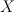$X$ be a nodal toric Fano threefold (recall that in toric world terminal Gorenstein singularities of Fano threefolds are simply ordinary double points aka nodes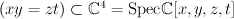$(xy=zt) \subset \CC^4 = \mathrm{Spec } \CC[x,y,z,t]$ ).

Given a terminal Gorenstein toric Fano threefold$X$,

this script do the following:

1. Compute Picard lattice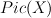$Pic(X)$
2. Then compute (self)intersection theory on this lattice.
This part is done in 3 steps:
a. pick a small crepant resolution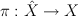$\pi : \hat{X} \rightarrow X$
b. compute intersection theory of smooth toric manifold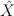$\hat{X}$,
c. restrict intersection theory from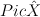$Pic \hat{X}$ to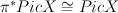$\pi^* Pic X \cong Pic X$.

3. Threefold X has a unique deformation class of smoothing by Fano threefold Y
We also compute the principal invariants of Y: Betti numbers, degree, Lefschetz discriminant and Fano index

The main procedure is called Picard(toric)
The input is a 3-component vector toric=[description, vertices, faces]
description is a verbal description of variety X (not used for computations)
vertices is a matrix of vertices of the fan polytope Delta(X)
faces is a transposed matrix of faces (vertices of the moment polytope)

The output is a 2-component vector o = [lattice, invariants]
where lattice is 3-component vector [cubic, M, class]
cubic is homogenous cubic polynomial of ‘rk Pic(X)’ variables (self-intersection pairing)
class is the expression of the first Chern class in terms of generators of Picard group
M is the matrix of the Lefschetz pairing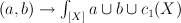$(a,b) \to \int_{[X]} a \cup b \cup c_1(X)$
and invariants is 5-component vector [rho,deg,b,d,r] of the principal invariants
rho is Picard number i.e. second Betti number of X
deg is the anticanonical degree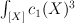$\int_{[X]} c_1(X)^3$
b is the half of third Betti number of the smoothing Y
d is the Lefschetz discriminant (i.e. determinant of matrix M)
r is the Fano index (i.e. divisibility of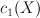$c_1(X)$ in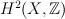$H^2(X,\ZZ)$)

## Surface mutations

I wrote a pari/gp script to do all the mutations in dimension 2 –
can mutate mirrors for all 10 del Pezzo surfaces.
By the way I found some typos (related to signs) in our paper.
See the file with the script for the correct formulas.

degmir.gp

## Quantum Lefshetz for non-split bundle via “abelian/non-abelian correspondence”.

Bumsig Kim explained me how their beautiful theory provides a tool for computing J-series of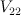$V_{22}$ and many other Fano threefolds.

The computation for$V_{22}$ can be reproduced by the following pari/gp code (I omit checking that mirror map is almost trivial):

 N=9 o = O(t^(N+1)) h(n) = sum(k=1,n,1/k) hh(n) = sum(k=1,n,sum(l=k+1,n,1/k/l)) gg(x, p) = (x!*(1+h(x)*p+hh(x)*p^2+O(u^4))) simplemirrormap(F) = F * exp(-polcoeff(F,1,t)*t) reg(F) = sum(n=0,N,t^n*polcoeff(F,n,t)*n!)+O(t^(N+1)) period(F) = reg(simplemirrormap(F))

 gp > v22 = period( polcoeff( sum(a=0,N,sum(b=0,N,sum(c=0,N,o+ t^(a+b+c)* ( gg(a+b,u*(A+B)) * gg(a+c,u*(A+C)) * gg(b+c,u*(B+C)) )^3 / (gg(a,u*A) * gg(b,u*B) * gg(c,u*C) )^7 *(c-b+u*(C-B))*(c-a+u*(C-A))*(b-a+u*(B-A)) ))) + O(u^4) , 3,u) /(C-B)/(C-A)/(B-A) + o ) 

%2 = 1 + 12*t^2 + 60*t^3 + 636*t^4 + 5760*t^5 + 58620*t^6 + 604800*t^7 + 6447420*t^8 + O(t^9) 
Indeed, period sequence 17.
Note that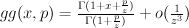$gg(x,p) = \frac{\Gamma(1 + x + \frac{p}{z})}{\Gamma(1 + \frac{p}{z})} + o(\frac{1}{z^3})$ is the familiar Gamma-factor with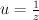$u=\frac{1}{z}$.

——————————————————-

So, how does it works?

Consider 3-dimensional vector space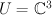$U = \CC^3$ with a fixed base, 7-dimensional vector space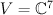$V = \CC^7$, and space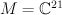$M = \CC^{21}$ of 3×7 matrices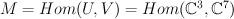$M = Hom(U,V) =Hom(\CC^3, \CC^7)$

Group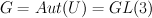$G = Aut(U) = GL(3)$ acts on M by left multiplication. It has a subgroup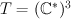$T = (\CC^*)^3$ of diagonal matrices and one may restrict the action to this smaller subgroup.

Let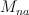$M_{na}$ be the subset of matrices of maximal rank and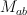$M_{ab}$ be the subset of matrices with non-vanishing rows,$M_{na}$ is an open subset in$M_{ab}$.

Consider quotient spaces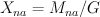$X_{na} = M_{na} / G$ and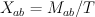$X_{ab} = M_{ab} / T$. Note that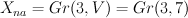$X_{na} = Gr(3,V) = Gr(3,7)$ and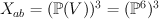$X_{ab} = (\PP(V))^3 = (\PP^6)^3$.

Since T is a subgroup of G, there is a natural rational map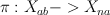$\pi: X_{ab} -> X_{na}$: a triple of points in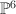$\PP^6$ is sent to their linear span.

Weyl group (symmetric group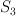$S_3$) acts on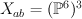$X_{ab} = (\PP^6)^3$ and hence it acts on the cohomology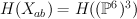$H(X_{ab}) = H((\PP^6)^3)$, so cohomology space is decomposed into representations of$S_3$.

[I’ll omit the part of the story with the partial flag space and non-holomorphic map].

1. It turns out that cohomology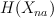$H(X_{na})$ can be identified with antisymmetric part of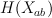$H(X_{ab})$ as a graded vector space (with grading shifted by 3).
Explicitly,$H(X_{ab})$ is generated by 3 pullbacks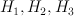$H_1, H_2, H_3$ of hyperplane sections on$\PP^6$; cohomologies of Grassmanian are known to be quotient of symmetric polynomials. Vector space of anti-symmetric polynomials is obtained from vector space of symmetric polynomials via multiplication by anti-symmetric polynomial of the smallest degree$Formula does not parse: \Delta = \prod_{i2.Also we can compare vector bundles on [latex]X_{ab}$ and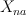$X_{na}$ by pulling them back to M, and considering as G-linearized.
It turns out that universal bundle U over Gr(3,7) decomposes into sum of 3 line bundles on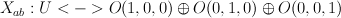$X_{ab}: U <-> O(1,0,0) \oplus O(0,1,0) \oplus O(0,0,1)$.
So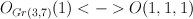$O_{Gr(3,7)}(1) <-> O(1,1,1)$ and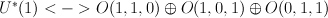$U^*(1) <-> O(1,1,0) \oplus O(1,0,1) \oplus O(0,1,1)$.

3. On domain of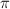$\pi$ one may define a relative tangent bundle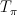$T_{\pi}$ (“traceless” part of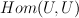$Hom(U,U)$). It turns out this vector bundle can be extended as a split vector bundle to whole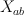$X_{ab}$: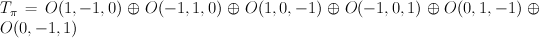$T_{\pi} = O(1,-1,0) \oplus O(-1,1,0) \oplus O(1,0,-1) \oplus O(-1,0,1) \oplus O(0,1,-1) \oplus O(0,-1,1)$.
Consider “square root” of relative tangent bundle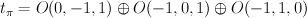$t_{\pi} = O(0,-1,1) \oplus O(-1,0,1) \oplus O(-1,1,0)$.

4.Recall that Fano threefolds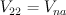$V_{22} = V_{na}$ are sections of homogeneous vector bundle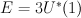$E = 3 U^*(1)$ on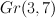$Gr(3,7)$. Comparision (2) shows these threefolds has 9-dimensional abelianizations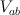$V_{ab}$ — complete intersections of $9$-dimensional split bundle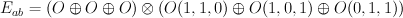$E_{ab} = (O \oplus O \oplus O) \otimes (O(1,1,0) \oplus O(1,0,1) \oplus O(0,1,1))$ on$X_{ab}$.

5. Abelian/non-abelian correspondence is similar for pairs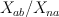$X_{ab}/X_{na}$ and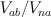$V_{ab}/V_{na}$.
J-series for Gr(3,7) can be obtained as twisted by relative tangent bundle$T_{\pi}$ I-series for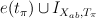$e(t_{\pi}) \cup I_{X_{ab},T_{\pi}}$ after the comparision of cohomologies described in (1).
Similarly, J-series for$V_{22}$ can be obtained via mirror map from twisted by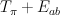$T_{\pi} + E_{ab}$ I-series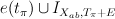$e(t_{\pi}) \cup I_{X_{ab},T_{\pi}+E}$ after the “pullbacked” comparision (1).

6. The sign comes from considering closely the Gamma-factor for relative tangent bundle$T_{\pi}$. Note that fibers of abelian/non-abelian correspondence are in some sense holomorphic symplectic (relative tangent bundle contains both O(D) and O(-D)), so they behave like varieties with trivial canonical class.
Consider the factor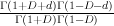$\frac{\Gamma(1+D+d) \Gamma(1-D-d)}{\Gamma(1+D)\Gamma(1-D)}$. Since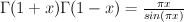$\Gamma(1+x) \Gamma(1-x) = \frac{\pi x}{sin (\pi x)}$ and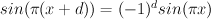$sin (\pi (x+d)) = (-1)^d sin(\pi x)$ we have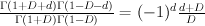$\frac{\Gamma(1+D+d) \Gamma(1-D-d)}{\Gamma(1+D)\Gamma(1-D)} = (-1)^d \frac{d+D}{D}$.

The same method can also be applied to complete intersections of homogeneous bundles in orthogonal isotropic and symplectic isotropic Grassmanians, since these Grassmanians themselves are just sections of some homogeneous bundles (wedge or symmetric powers of universal bundle) on ordinary Grassmanians of type A. Also this can be uprgaded to treat different blowups of these varieties. In particular, in the comments to this post I compute J-series for Fano threefolds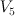$V_5$, #2.14, #2.17, #2.20, #2.21 and #2.22.

Also Bumsig points out that one can express the ab/non-ab twist as a differential operator applied to abelian multi-parameter J-function (basically, just Vandermonde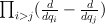$\prod_{i>j} (\frac{d}{d q_i} - \frac{d}{d q_j})$. This interpretation is more useful for dealing with Frobenius manifolds.

References:
Gromov-Witten Invariants for Abelian and Nonabelian Quotients by Aaron Bertram, Ionut Ciocan-Fontanine, Bumsig Kim
The Abelian/Nonabelian Correspondence and Frobenius Manifolds by Ionut Ciocan-Fontanine, Bumsig Kim, Claude Sabbah
Quantum cohomology of the Grassmannian and alternate Thom-Sebastiani by Bumsig Kim, Claude Sabbah